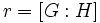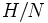Union of all conjugates of subgroup of finite index is proper

This article describes an easy-to-prove fact about basic notions in group theory, that is not very well-known or important in itself
View other elementary non-basic facts
VIEW FACTS USING THIS: directly | directly or indirectly, upto two steps | directly or indirectly, upto three steps|

This article describes a result of the form that argues that a subset constructed in a certain fashion is proper, viz it is not the whole group

Statement

Verbal statement

The union of conjugates of a proper subgroup of finite index in a group, is a proper subset. In particular, in a finite group, the union of all conjugates of any proper subgroup is a proper subset.

Symbolic statement

Let$H$ be a proper subgroup of finite index in a group$G$. Then, the union of all conjugates of$H$ is a proper subset of$G$. In other words:$\bigcup_{g \in G} gHg^{-1} \ne G$

Property-theoretic statement

Call a subgroup conjugate-dense if the union of its conjugates is the whole group. Then, the result states that:

conjugate-dense$\land$ subgroup of finite index$\implies$ whole group (viz improper subgroup)

Related facts

Breakdown for infinite groups

If$G$ is infinite and$H$ is a subgroup of infinite index,$H$ may be conjugate-dense in$G$: every element of$G$ may be conjugate to an element of$H$. Examples include upper triangular matrices in the general linear group over an algebraically closed field. For more information, see:

Related notion of contranormality

Note that the result only says that the union of the conjugates must be a proper subset. It does not state that the subgroup generated by the union of the conjugates must be a proper subgroup. The subgroup generated by the union of the conjugates of a proper subgroup, which is also called its normal closure, may well be the whole group. A subgroup wholse normal closure is the whole group is termed a contranormal subgroup.

Any conjugate-dense subgroup is contranormal, but while no proper subgroup of finite index can be conjugate-dense, there can exist contranormal proper subgroups of finite index (for instance, any maximal non-normal subgroup).

Facts used

1. Fundamental theorem of group actions (also see Group acts on set of subgroups by conjugation for an explanation of the application of the fundamental theorem of group actions to this concrete situation).
2. Lagrange's theorem: This states that for$H \le G$, we have$|G| = |H|[G:H]$.
3. Poincare's theorem: Any subgroup of finite index in a group contains a normal subgroup of finite index.
4. Fourth isomorphism theorem: The correspondence between subgroups containing a normal subgroup and subgroups in the quotient group.

Proof

Finite case

Given: A finite group$G$ of order$n$, and a subgroup$H$ of index$r > 1$.

To prove: The union of conjugates of$H$ in$G$ is a proper subset of$G$.

Proof: We proceed as follows::

1. The index$s = [G:N_G(H)]$ is bounded by the index$r = [G:H]$:$N_G(H)$ (the normalizer of$H$ in$G$) is a subgroup of$G$ containing$H$. Thus, the index (say$s$) of$N_G(H)$ in$G$ is at most$r$.
2. The number of conjugate subgroups to$H$ in$G$ equals$s$: Consider the action of$G$ on the set of conjugate subgroups to$H$ by conjugation. The stabilizer of$H$ is precisely$N_G(H)$, so by fact (1), there is a bijection between the cosets of$N_G(H)$ in$G$ and the conjugate subgroups to$H$ in$G$. Thus, the number of conjugate subgroups to$H$ in$G$ equals$s = [G:N_G(H)]$.
3. Since all the conjugates have equal size and already intersect in the identity element, the total number of elements in the union is at most$1 + s(|H| - 1)$. We bound this as follows:$1 + s(|H| - 1) \le 1 + r(|H| - 1) = 1 + r|H| - r = 1 + [G:H]|H| - r = |G| - (r - 1) < |G|$

(An intermediate step uses Lagrange's theorem, and the final step uses the fact that$r > 1$).

Thus, the union of all conjugates of$H$ in$G$ is a proper subset of$G$.

Case of finite index

Given: A group$G$, a subgroup$H$ of finite index in$G$.

To prove: The union of all conjugates of$H$ in$G$ is a proper subset of$G$.

Proof:

1. By fact (3), there is a normal subgroup$N$ of finite index in$G$ contained inside$H$. Let$\varphi:G \to G/N$ be the quotient map. Thus,$G/N$ is a finite group and$H/N$ is a proper subgroup of$G/N$.
2. By fact (4) (the fourth isomorphism theorem) or a direct checking, we see that the conjugates of$H$ in$G$ are precisely the inverse images under$\varphi$ of the conjugates of$H/N$ in$G/N$.
3. Applying the result in the finite case to the group$G/N$ and the proper subgroup$H/N$, we obtain that the union of all conjugates of$H/N$ in$G/N$ is a proper subset of$G/N$. Combining this with step (2) yields that the union of all conjugates of$H$ in$G$ is a proper subgroup of$G$.